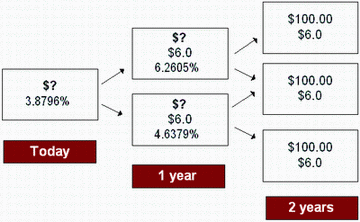### CFA Practice Question

There are 147 practice questions for this study session.

### CFA Practice Question

What is the value of a 2-year, 6% coupon, option-free bond given the following interest rate tree?A. 99.755
B. 101.302
C. 102.560
Explanation: Value of the bond at upper node for period 1 = [(100 + 6) / (1.062605) + (100 + 6) / (1.062605)] / 2 = 99.755.

Value of the bond at lower node for period 1 = [(100 + 6) / (1.046379) + (100 + 6) / (1.046379)] /2 = 101.302.

Current value of the bond at node 0 (today) = [(99.755 + 6) / (1.038796) + (101.302 + 6) / (1.038796)] /2 = 102.560.